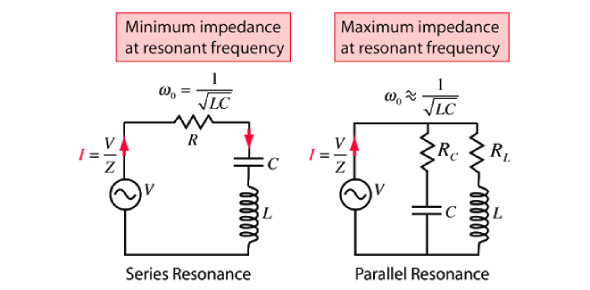# Quiz On power And Resonance In AC Circuits by Stan gibilisco

20 Questions | Attempts: 643
ShareSettingsMCQ in Power and Resonance in AC circuits by Stan Gibilisco forms part of every engineering syllabus. This quiz contains various questions that cover the vast aspects of the topic that not only gauges your knowledge but also provides you with valuable feedback that would help you in mastering the topic. The quiz would be very hand if you have an upcoming exam. If you like the quiz, do share it with your friends. All the best!

• 1.
The power in a pure reactance is
• A.

• B.

True

• C.

Imaginary

• D.

Apparent

• 2.
Which of the following is not an example of true power?
• A.

Power in the form of heat, produced by dc flowing through a resistor

• B.

Power in the form of electromagnetic fields, radiated from a radio antenna

• C.

The product of the rms ac through a capacitor and the rms voltage across it

• D.

Power in the form of heat, produced by losses in an RF transmission line

• 3.
Suppose the apparent power in a circuit is 100 W, and the imaginary power is 40 W. What isthe true power?
• A.

92 W

• B.

100 W

• C.

140 W

• D.

It is impossible to determine from this information

• 4.
Power factor is equal to
• A.

Apparent power divided by true power

• B.

Imaginary power divided by apparent power

• C.

Imaginary power divided by true power

• D.

True power divided by apparent power

• 5.
Suppose a circuit has a resistance of 300 Ω and an inductance of 13.5 µH in series, and isoperated at 10.0 MHz. What is the power factor?
• A.

0.334

• B.

0.999

• C.

0.595

• D.

It cannot be determined from the information given.

• 6.
Suppose a series circuit has Z = 88.4 Ω, with R = 50.0 Ω. What is the power factor, expressed as a percentage?
• A.

99.9 percent

• B.

56.6 percent

• C.

60.5 percent

• D.

29.5 percent

• 7.
Suppose a series circuit has R = 53.5 Ω, with X = 75.5 Ω. What is the power factor, expressed as a percentage?
• A.

70.9 percent

• B.

81.6 percent

• C.

57.8 percent

• D.

63.2 percent

• 8.
The phase angle in an ac circuit is equal to
• A.

Arctan (Z/R)

• B.

Arctan (R/Z)

• C.

Arctan (R/X)

• D.

Arctan (X/R)

• 9.
Suppose an ac ammeter and an ac voltmeter indicate that there are 220 W of VA power in acircuit that consists of a resistance of 50 Ω in series with a capacitive reactance of −20 Ω. What is the true power?
• A.

237 W

• B.

204 W

• C.

88.0 W

• D.

81.6 W

• 10.
Suppose an ac ammeter and an ac voltmeter indicate that there are 57 W of VA power in acircuit. The resistance is known to be 50 Ω, and the true power is known to be 40 W. What is the absolute-value impedance?
• A.

50 Ω

• B.

57 Ω

• C.

71 Ω

• D.

It is impossible to determine on the basis of this data.

• 11.
Which of the following should be minimized in an RF transmission line?
• A.

• B.

• C.

The line loss

• D.

The transmitter power

• 12.
Which of the following does not increase the loss in a transmission line?
• A.

Reducing the power output of the source

• B.

Increasing the degree of mismatch between the line and the load

• C.

Reducing the diameter of the line conductors

• D.

Raising the frequency

• 13.
Which of the following is a significant problem that standing waves can cause in an RFtransmission line?
• A.

Line overheating

• B.

Excessive power loss

• C.

Inaccuracy in power measurement

• D.

All of the above

• 14.
Suppose a coil and capacitor are in series. The inductance is 88 mH and the capacitance is1000 pF. What is the resonant frequency?
• A.

17 kHz

• B.

540 Hz

• C.

17 MHz

• D.

540 kHz

• 15.
Suppose a coil and capacitor are in parallel, with L = 10.0 µH and C = 10 pF. What is fo?
• A.

15.9 kHz

• B.

5.04 MHz

• C.

15.9 MHz

• D.

50.4 MHz

• 16.
Suppose you want to build a series-resonant circuit with fo = 14.1 MHz. A coil of 13.5 µH isavailable. How much capacitance is needed?
• A.

0.945 µF

• B.

9.45 pF

• C.

94.5 pF

• D.

945 pF

• 17.
Suppose you want to build a parallel-resonant circuit with fo = 21.3 MHz. A capacitor of22.0 pF is available. How much inductance is needed?
• A.

2.54 mH

• B.

254 µH

• C.

25.4 µH

• D.

2.54 µH

• 18.
A 1⁄4-wave section of transmission line is cut for use at 21.1 MHz. The line has a velocityfactor of 0.800. What is its physical length in meters?
• A.

11.1 m

• B.

3.55 m

• C.

8.87 m

• D.

2.84 m

• 19.
What is the fourth harmonic of 800 kHz?
• A.

200 kHz

• B.

400 kHz

• C.

3.20 MHz

• D.

4.00 MHz

• 20.
Suppose you want to build a 1⁄2-wave dipole antenna designed to have a fundamentalresonant frequency of 3.60 MHz. How long should you make it, as measured from end to end in feet?
• A.

130 ft

• B.

1680 ft

• C.

39.7 ft

• D.

515 ft

## Related TopicsBack to top
×

Wait!
Here's an interesting quiz for you.